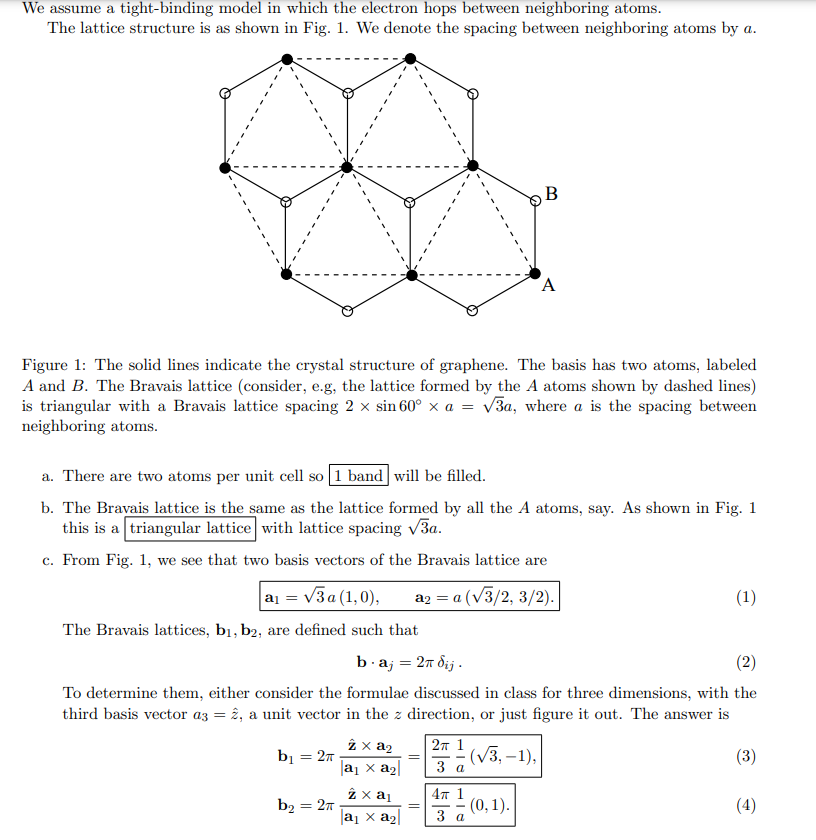# (Solved):   Please go through question c) in detail. I have no idea how to find bravais lattice ...Please go through question c) in detail.

I have no idea how to find bravais lattice vectors a1 a2 b1 and b2.

We assume a tight-binding model in which the electron hops between neighboring atoms. The lattice structure is as shown in Fig. 1. We denote the spacing between neighboring atoms by $$a$$. Figure 1: The solid lines indicate the crystal structure of graphene. The basis has two atoms, labeled $$A$$ and $$B$$. The Bravais lattice (consider, e.g, the lattice formed by the $$A$$ atoms shown by dashed lines) is triangular with a Bravais lattice spacing $$2 \times \sin 60^{\circ} \times a=\sqrt{3} a$$, where $$a$$ is the spacing between neighboring atoms. a. There are two atoms per unit cell so $$\quad$$ will be filled. b. The Bravais lattice is the same as the lattice formed by all the $$A$$ atoms, say. As shown in Fig. 1 this is a with lattice spacing $$\sqrt{3} a$$. c. From Fig. 1, we see that two basis vectors of the Bravais lattice are $\mathbf{a}_{1}=\sqrt{3} a(1,0), \quad \mathbf{a}_{2}=a(\sqrt{3} / 2,3 / 2) .$ The Bravais lattices, $$\mathbf{b}_{1}, \mathbf{b}_{2}$$, are defined such that $\mathbf{b} \cdot \mathbf{a}_{j}=2 \pi \delta_{i j} .$ To determine them, either consider the formulae discussed in class for three dimensions, with the third basis vector $$a_{3}=\hat{z}$$, a unit vector in the $$z$$ direction, or just figure it out. The answer is $\begin{array}{l} \mathbf{b}_{1}=2 \pi \frac{\hat{\mathbf{z}} \times \mathbf{a}_{2}}{\left|\mathbf{a}_{1} \times \mathbf{a}_{2}\right|}=\frac{2 \pi}{3} \frac{1}{a}(\sqrt{3},-1), \\ \mathbf{b}_{2}=2 \pi \frac{\hat{\mathbf{z}} \times \mathbf{a}_{1}}{\left|\mathbf{a}_{1} \times \mathbf{a}_{2}\right|}=\frac{4 \pi}{3} \frac{1}{a}(0,1) . \end{array}$

We have an Answer from Expert